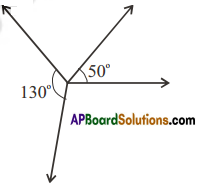AP State Syllabus AP Board 7th Class Maths Solutions Chapter 4 Lines and Angles Ex 5 Textbook Questions and Answers.

## AP State Syllabus 7th Class Maths Solutions 4th Lesson Lines and Angles Exercise 5Question 1.
Draw the following pairs of angles as adjacent angles. Check whether they fonn linear pair.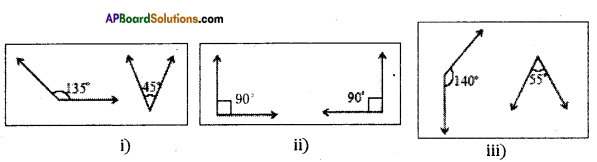Solution:
Linear pair of angles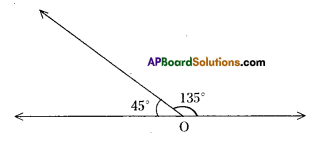Linear pair of angles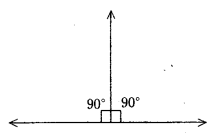Do not form linear pair.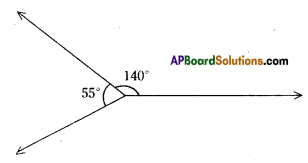Question 2.
Niharika took two angles- 1300 and 500 and tried to check whether they form a linear pair. She made the following picture.
Solution:
Yes. These two angles do not form a pair of linear angles.
Because she made angle more than 180°.